# Class 6 Maths Basic Geometrical Ideas WOrksheet

In this page we have Class 6 Maths Basic Geometrical Ideas Worksheet. Hope you like them and do not forget to like , social share and comment at the end of the page.
Topic of Circles,Triangles and Quadrilaterals is deleted from this chapter as per rationalized syllabus.So you may ignore those Questions
Question 1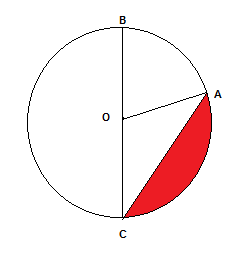(a) Write the Diameter in the figure
(b) Write the radius in the figure
(c) Write the chord in the figure
(d) What is the red portion called
(e) BC = ___ OC

Question 2
Which is of these is not a polygon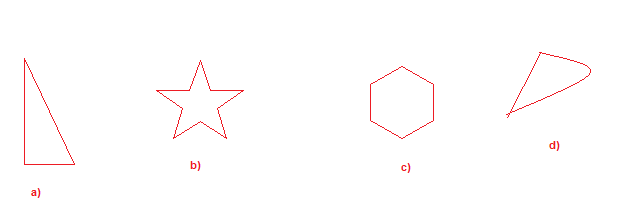Question 3
Which is of these is not a closed curve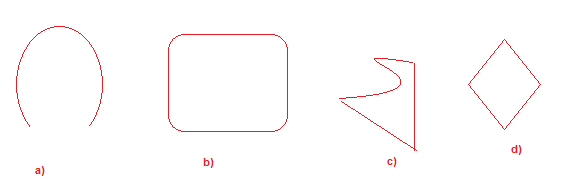Question 4
Which is of these is not a simple curve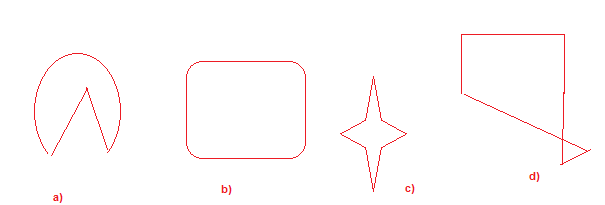Question 5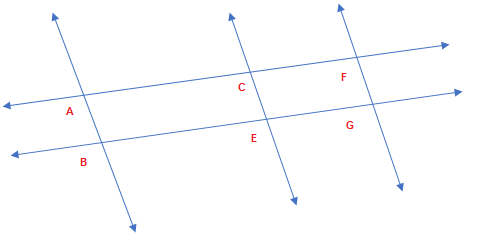Please find below based on above figure
(a) A pair of intersecting lines
(b) A pair of parallel lines
(c) Intersecting points
(d) Write down two line segments in the figure
(e) Represent all the lines in the figure
Question 6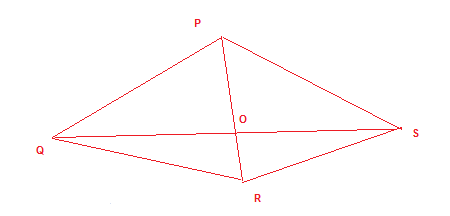(a) Find all the triangles in the above figures
(b) Find all the angles in the figure
(1)
(a)BC
(b) OB,OC,OA
(c) Ac and BC
(d) segment
(e) 2
2) (d) 3) (a) 4) (d)

• Notes and NCERT Solutions
• Assignments### Practice Question

Question 1 What is $\frac {1}{2} + \frac {3}{4}$ ?
A)$\frac {5}{4}$
B)$\frac {1}{4}$
C)$1$
D)$\frac {4}{5}$
Question 2 Pinhole camera produces an ?
A)An erect and small image
B)an Inverted and small image
C)An inverted and enlarged image
D)None of the above